Study Guide

You might be wondering how Expo and Log got together in the first place. It couldn't just be Expo's charming good looks, right? In this section, you're going to learn all about how logarithms and exponents are connected—how they're the same and how they're total opposites of one another.

### What's a Log, Anyway?

Before we get into the nitty-gritty of how logs work, let's take a gander at how they look. Here's one:

log10x

Weird, right? Why is there a number below the log? What's the log doing to the x? Sometimes you've got to know what's inside to know how something works. Just like in biology class, except as we cut into exponents and logarithms it won't be nearly as squishy or disgusting. We promise.

### Inverse Operations

First and foremost, you've got to know that logarithms are inverse operations of exponentials. Yup, we're turning your world upside-down. Please rotate your monitor around so that you can read the rest of this section. If you can't, you'll just have to get used to reading everything that way. Unfortunately, we've run out of hot towels for all the sore necks caused by this new policy, so you'll need to provide those yourself.Mathematics gets stuff done through operations. Otherwise we'd have a bunch of values and nothing to do with them; we'd have no way to connect them. Enter operations.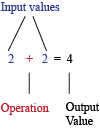Operations string values together to produce other values. However, sometimes we need stuff undone, so we created inverse operations. Inverse operations are mathematical takesy backsies: they're operations that undo one another. Addition and subtraction are inverse operations of each other. Multiplication and division are also inverse operations of each other. If you started with 3, multiplied it times 2, but then divided the answer by 2, you'd be right back to 3.

Guess where we're going with this? Exponents and logarithms are inverse operations! So if we have this equation:

y = x

We can add to both sides…

y + 2 = x + 2

We can subtract from both sides…

y – 2 = x – 2

Multiply…

2y = 2x

Divide…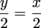You can also "exponentiate" both sides:

10y = 10x

And finally "take the log" of both sides:

log y = log x

Every single one of the expressions here are equivalent to y = x and to each other. Because you know that logs and exponents are inverse operations, just like addition and subtraction are, manipulating equations with exponents will be a snap.

Now that you know exponentiating and taking the log are inverse operations, let's talk inverse functions.

### Inverse Functions

Imagine a function that has this set of inputs and outputs. No really, close your eyes and focus on your inner mathlete:

{(1, 5), (2, 9), (3, 13), (4, 17)}

If you're on the up and up, you might recognize these numbers come from the linear function y = 4x + 1. Awesome. The inverse of this function simply switches all of the input and output values:

{(5, 1), (9, 2), (13, 3), (17, 4)}

What's that function? No cheating, we've got robots working for us.

A very simple way of figuring out the answer to this question is to solve for x and switch the x and y.

y = 4x + 1
y 1 = 4x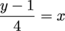Then, we switch the x and the y.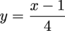Bam! There's your answer:is the inverse of y = 4x + 1. A clearer way to represent this would be to say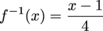.

Special note: f -1(x), the inverse function, is not the same as the reciprocal function,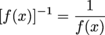. Be careful!

If you applied a function and its inverse function to an input value, the input would come out of the works unchanged. Prove this to yourself: try throwing a 4 into either function and then put the answer in the other.

See? It's still 4. (If not, something has gone terribly wrong and this message will self-destruct in 3 seconds.) In other, more math-y terms:

f -1[f(x)] = x

Here's some proof:

Step 1, The Awesome Step: y = 4(4) +1 = 17

Step 2, The Awesomer Step: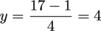Impressed yet? It's like a magician who walks on the stage, pulls a rabbit out of the hat, and…WHOA THAT'S NOT A RABBIT! He quickly puts the non-rabbit back into the hat and walks backwards off the stage as if nothing happened. No refunds.In the next section, we'll try to find the inverse of an exponential function. It's not going to be quite as easy as this section, but you'll feel even awesomer-er when you figure things out.

### Inverses of Exponential Functions

Apply the magical lesson of the inverse to an exponential function. First, take a look at an exponential function. Like we mentioned before, exponential functions are simpler than they look: just stick the x in the exponent.

Here's one:

y = 10x

Right. So what about its inverse? Just solve for x. Wait—how is this supposed to work?

Without logarithms, you might be stuck in the mud. Dirty, goopy, nasty mud that won't wash out no matter how many times you put it in the washer. Math can do anything; it can even keep your clothes clean and fresh. (Disclaimer: Do not attempt to put math in the washer; this will void all warranties.)

Go along with us for a second here. We're going to use a logarithm to solve for x and then switch things around. Like we said in the last section, taking the log is the inverse operation of exponentiation, so we can use it to cancel the 10 on the right side of the equation. Start by taking the log of both sides:

log10 y = log10 10x

log10 y = x

Now we swap x and y to get our inverse function:

log10 x = y

y = log10 x

Ta-daaa: y = log10 x, a logarithmic function, is the inverse of y = 10x, an exponential function

• ### Rules for Inverse Functions

There are a few rules for whether a function can have an inverse, though. Even though you can buy anything you want in life, a function doesn't have the same freedoms in math-life.

First of all, it's got to be a function in the first place. For a review of that, go here...or watch this video right here:

Second, that function has to be one-to-one. That is, for every x-value, there's got to be a unique y-value.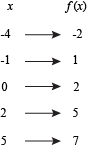This is a one-to-one function.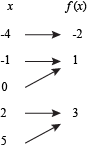This is not. Notice how multiple x-values can yield the same y-value.

Figuring out if a function is one-to-one is as simple as drawing a straight line. No, really—give it a shot. It's called the horizontal line test. Draw any function, and then draw a straight horizontal line through it. If there's anywhere that the line passes through the function more than once, it's not a one-to-one function, which means it doesn't have an inverse.

This is a bit like the vertical line test that we used to figure out if a relation counts as a function. The vertical line test tells us if we have a function, and the horizontal line test tells us if our function has an inverse. Got it?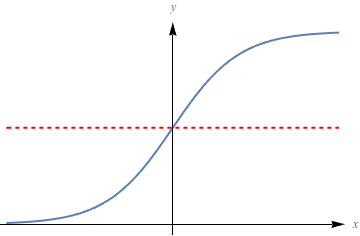This function passes the horizontal line test, so it's got an inverse.

### Sample Problem

Which of the following is not a one-to-one function? Try drawing them if you have trouble.

a. f(x) = x2 + 4
b. f(x) = -4x
c. f(x) = 2x

The answer is a. Because this function is even, or symmetric across the y-axis, the horizontal line test fails, and it is not one-to-one. Don't worry, the function won't be punished. It's just part of a different circle of friends. It still counts as function, but it has no inverse.

If a function isn't one-to-one, though, there's a simple way to make it conform: remove the parts that fail the horizontal line test. For example, if we have the function x2 + 4 from the sample problem: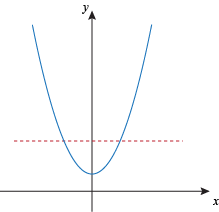This function fails the horizontal line test. No re-takes either—bummer.

All we've got to do is restrict the domain of the function so that it does pass the test with flying colors.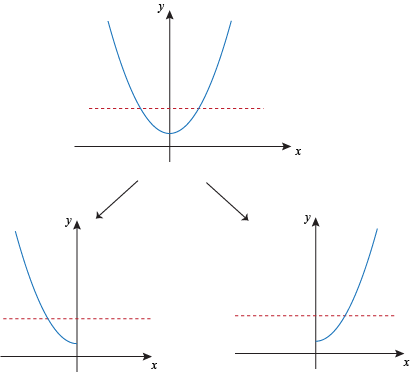Here we restrict the domain to either x < 0 or x > 0, and now it's one-to-one.

### Translating Logs

Now that we know about how exponents and logarithms are inverse operations of each other, it's time to explore the deep, dark caverns of the base. Take a look at the function we inverted before:

y = 10x
log10 y = x

The first thing to pop out like a jack-in-a-box is that 10, the base of the exponent, is now attached below the log. Guess what? It's also called the base: the base of the logarithm.

So where's the exponent?

It's still the x. Bingo. It's one and the same.

That means the entire expression log10 y is the exponent you need to raise the base 10 to so you get y. For example, what is log10 100? To figure this out, ask yourself the following question: 10 raised to the power of what would give 100? The answer is 2, because 102 = 100.

log10 100 = 2

Logarithms are just another way of writing down exponents. The equations y = 10x and log10 y = x are completely equivalent to each other.

Here's an illustration showing how logs and exponents are related: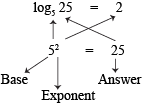In fact, we can represent any exponential in log form and vice versa.

### Sample Problem

What is log4 64?

If you look at the base, it's 4. So, what is the exponent needed to give us an answer of 64? Let's try sticking a few exponents on a base of 4.

41 = 4
42 = 16
43 = 64

Ah, there we go: 43 = 64, so log64 = 3.

You may have noticed before that we used a logarithm to simplify the following equation:

log10 y = log10 10x
log10 y = x

The reason log1010x = x is because the exponent you need to raise 10 to so you get 10x is x. This can be used for any other base:

logb bx = x

And it works the other way, too. If we have a number raised to a log whose base is that same number, they cancel out:

blogb x = x

A log can have a base of any positive number, any one you like. It's also possible to have a log with a negative base, but they're mean, nasty, and don't even help clean up after eating dinner. You can safely avoid them.

Logarithms always have a base, just like those couples that just can't get away from each other. If you see a logarithm written without a base, don't panic. It's not lonely; the base just has an invisibility cloak. Which base does it have? Good ol' number 10.

log x = log10 x

A base-10 log even has a special name: the common logarithm.

### Sample Problem

Solve y = 42x for x.

Now that we know all logs have a base, and they can have a base of any positive number, let's throw a log with a base of 4 on both sides of our equation.

y = 42x

logy = log42x

The stuff on the right side is asking, "What exponent do we need to stick on a base of 4 to get an answer of 42x ?" The answer is just 2x. In other words, the log4 cancels out the 4 and leaves us with the exponent.

log4 y = 2x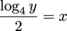• ### The Natural Log

Another special type of logarithm you'll see from time to time is the natural logarithm, usually written as ln(x) instead of log(x). We like to think the "n" comes after the "l" because it stands for log au naturel.

A natural log is the same as a regular log, except it has a base of e instead of 10. If you haven't met him before, e is an irrational number that represents the sum of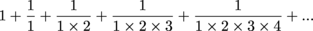and so on.

Weird, right? But it's really useful! You'll see the natural log all the time in higher-level math, but we'll show you a common use in a later chapter. For now, just remember this:

ln x = loge xTo confuse things, some people write ln x as loge x or even log x. We wouldn't do that; keep an eye out, though!